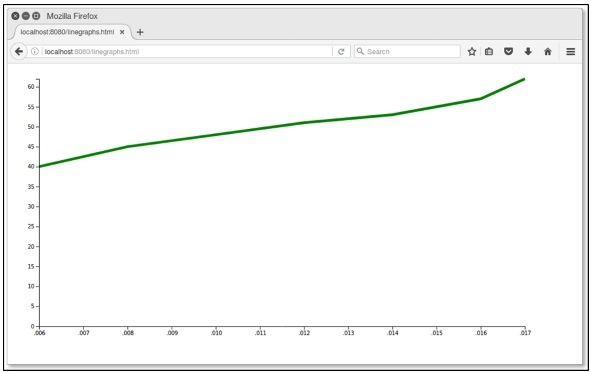# D3.js - 图表

## SVG 坐标空间

• SVG 坐标空间的 x = 0，y = 0 坐标位于左上角。
• SVG 坐标空间的 Y 坐标从上到下增长。

### SVG 坐标空间图

var svgContainer = d3
.select("body")
.append("svg")
.attr("width", 200)
.attr("height", 200);


var svgContainer = d3
.select("body").append("svg")
.attr("width", 200)
.attr("height", 200)
.style("border", "1px solid black");


### 图示例

year,population
2006,40
2008,45
2010,48
2012,51
2014,53
2016,57
2017,62


.line {
fill: none;
stroke: green;
stroke-width: 5px;
}


var margin = {top: 20, right: 20, bottom: 30, left: 50},
width = 960 - margin.left - margin.right,
height = 500 - margin.top - margin.bottom;


var valueline = d3.line()
.x(function(d) { return x(d.year); })
.y(function(d) { return y(d.population); });


var svg = d3.select("body").append("svg")
.attr("width", width + margin.left + margin.right)
.attr("height", height + margin.top + margin.bottom)
.append("g").attr("transform",
"translate(" + margin.left + "," + margin.top + ")");


d3.csv("data.csv", function(error, data) {
if (error) throw error;
}


data.forEach(function(d) {
d.year = d.year;
d.population = +d.population;
});


x.domain(d3.extent(data, function(d) { return d.year; }));
y.domain([0, d3.max(data, function(d) { return d.population; })]);


svg.append("path").data([data])
.attr("class", "line").attr("d", valueline);


svg.append("g")
.attr("transform", "translate(0," + height + ")")
.call(d3.axisBottom(x));


svg.append("g")
.call(d3.axisLeft(y));


graph.html

<!DOCTYPE html>
<html>
<script type = "text/javascript" src = "https://d3js.org/d3.v4.min.js"></script>
<style>
.line {
fill: none;
stroke: green;
stroke-width: 5px;
}
</style>

<body>
<script>
// set the dimensions and margins of the graph
var margin = {top: 20, right: 20, bottom: 30, left: 50},
width = 960 - margin.left - margin.right,
height = 500 - margin.top - margin.bottom;

// set the ranges
var x = d3.scaleTime().range([0, width]);
var y = d3.scaleLinear().range([height, 0]);

// define the line
var valueline = d3.line()
.x(function(d) { return x(d.year); })
.y(function(d) { return y(d.population); });

// append the svg obgect to the body of the page
// appends a 'group' element to 'svg'
// moves the 'group' element to the top left margin
var svg = d3.select("body").append("svg")
.attr("width", width + margin.left + margin.right)
.attr("height", height + margin.top + margin.bottom)
.append("g").attr("transform",
"translate(" + margin.left + "," + margin.top + ")");

// Get the data
d3.csv("data.csv", function(error, data) {
if (error) throw error;
// format the data
data.forEach(function(d) {
d.year = d.year;
d.population = +d.population;
});

// Scale the range of the data
x.domain(d3.extent(data, function(d) { return d.year; }));
y.domain([0, d3.max(data, function(d) { return d.population; })]);

svg.append("path")
.data([data])
.attr("class", "line")
.attr("d", valueline);

svg.append("g")
.attr("transform", "translate(0," + height + ")")
.call(d3.axisBottom(x));